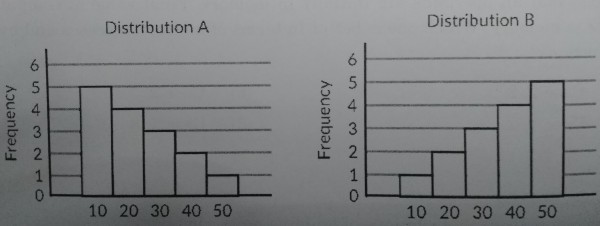Open in App
Not now

# ISRO | ISRO CS 2020 | Question 39

• Last Updated : 04 Sep, 2020

For the distributions given below :Which of the following is correct for the above distributions ?
(A) Standard deviation of A is significantly lower than standard deviation of B
(B) Standard deviation of A is slightly lower than standard deviation of B
(C) Standard deviation of A is same as standard deviation of B
(D) Standard deviation of A is significantly higher than standard deviation of B